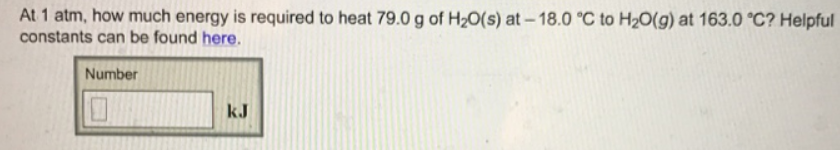Chemistry Calorimetry Solution: At 1 atm, how much energy is required to heat 79.0...

🤓 Based on our data, we think this question is relevant for Professor Bhaduri's class at Saint Marys College of California.

# Solution: At 1 atm, how much energy is required to heat 79.0 g of H 2O(s) at -18.0°C to H 2O(g) at 163.0°C? Helpful constants can be found here.

Problem

At 1 atm, how much energy is required to heat 79.0 g of H 2O(s) at -18.0°C to H 2O(g) at 163.0°C? Helpful constants can be found here.View Complete Written Solution